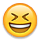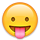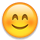Normalizing Solar Array Efficiency

Is this possible?
Given hourly data on kW(instantaneous) tilt, rated output, calculate the efficiency of an array as if it were at a 0 degree angle.

Hello Everyone,
I am an Electrical Engineering student at the University of Cincinnati and I am currently on co-op. I have been tasked with normalizing the efficiency of several different PV arrays which are all at different constant angles.
In other words, if array A is at an angle of 35 degrees and has an efficiency of 35%. What is the efficiency of the array at 0 degrees? (these are just random numbers of course)
I have done a LOT of looking around for a simple solution online and it seems that it does not exist, so I have attempted to create one myself.

What I did:
I wrote a program (in C++ because that is the only language I know) and a table in excel which could calculate the Solar Radiation incident on a surface at any angle given : Day of the year, hour of the day, tilt angle, and latitude. (assuming 1000W/m^2)

I used my program, and excel to simulate data for approximately a month from the end of December to the middle of January.

and averaged this value over the 20ish days.
and recorded the value at times of: 8:00AM to 4:00 PM (the sun sets around 5 in southern Ohio in the winter so the data becomes negative as does the solar declination)
Soooo... Averaging the ratios of the individual hours gives me the average hour during the day and the average ratio at that hour. (using best line fit the data shows the pattern of an upside down parabola for Hour v. Tilt Angle)

Recap, I now have an average ratio from the time period of Dec. 25th to Jan. 18 of the Solar radiation between a tilted 25 degree angle, and a horizontal zero degree angle surface. This will serve as the correction factor for the 25 degree surface.

The company I work for has provided me with some measurements, including instantaneous kW output of each array every hour over the given time period above. I also have the rated output for each array. SO......
After calculating the average kWh of each array,
I take----> (Average kWh)/(Rated kW)*(Correction Factor)
The average kWh data excludes 0 data or data less than 10Wh.

This is my approach, any comments or constructive criticisms are welcome.

Correction Factors:(Based on Latitude 39 degrees)
Tilt Correction Factor
35 0.3977
40 0.3748
10 0.673
25 0.465
7.4 0.7359

Example Calculation (Array is at 25 degrees)

Roof Ground
Average kWh: 0.8708 2.7381 (From the measured and recorded data)
Corrrected for tilt: 1.26 (correction factor of .465)
Ratio corrected: 69.31% (what percentage of the kWh the roof
array is producing compared to the ground array)
Ratio not corrected: 31.80%

• Banned Posts: 17,615 ✭✭
Re: Normalizing Solar Array Efficiency

I have to admit I'm not sure what you're after but ... There's quite a few factors involved in determining the efficiency of any given array.

#1 Angle. Why do you want to use zero degrees? Panels need to be "parallel" with the sun to peak their output. A good explanation found here: http://www.macslab.com/optsolar.html

#2 Temperature. Panel heats up, efficiency goes down. This is not just the question of where in the world it's located either; anywhere the panel temp will rise as the sun shines on it.

#3 Altitude and other atmospheric conditions. The clearer the sky, the better of course. My system over-all runs about 5% better than typical because it's at 3,000+ feet.

#4 Wiring. Long runs, small wires drop the available output.

Again I'm not sure what you're trying to accomplish exactly. Sounds like an academic exercise and I tend more towards practical application. Crystaline panels in general are only about 18% efficient at best. If somebody could do something about that ...

(Note: if this is completely off base blame the ketorolac I'm trying to function with. A bit loopy. Sorry.)
• Re: Normalizing Solar Array Efficiency

It seems that this is more of a 'calculation' problem, and not so much practical, so if you don't reply I wont blame you.I apologize if I was not specific enough in my post as to what exactly I am trying to do. The company that I work for is trying to compare the efficiencies of several different PV modules which they currently have set up at the headquarters in OH. The problem is:
They are all at different tilt angles, and the tilt angles can not be changed easily. Thus they are rigid and fixed.
So the trick (I think) is to figure out the relationship between the tilt angle and the kWh production of the array, and lets say another array which is at 0 degrees. I chose 0 because it seemed like a decent standard value. (we could have just as easily chose the latitude of the location, my hypothesis is that the relationship should be constant).
Obviously the array at a tilt will produce more kWh in the winter due to the sun being lower in the sky. (I have done solar radiance calculations also to show this)
So the solution would have to correct the tilted array to show the production as if it were at a 0 degree angle.
Re: Normalizing Solar Array Efficiency

Between different orientations, possible weather impact during the day, etc... I don't see a way of comparing panels with better than 10% accuracy by doing the math.

Your options are to use a solar program to predict average output against measured output peer panel or array of same panels...

Or use a data logger to gather data. One logger per panel orientation each with an identical reference panel.

http://www.microcircuitlabs.com/SDL-1.htm

But even a simple device like above is only +/- 5% accurate.

Are you looking for "good" panels (80% or more of rated output) vs "bad"panels (less than 80%)?

-Bill
Near San Francisco California: 3.5kWatt Grid Tied Solar power system+small backup genset
• Banned Posts: 17,615 ✭✭
Re: Normalizing Solar Array Efficiency

Yes I can see the problem, even through my hazy reasoning.

You've got too many variables, can't "suspend" any of them, and are introducing another (the zero angle theoretical panel).

From a practical point of viewI'd suggest you get an "ideal" angle from the Mac's lab calculator and compare to that. Since it's all relative, find whichever panel is closest to "ideal", determine its output individually (may be necessary to install some additional metering), and get a "typical" derating factor.

Something like this (all theoretical):

Best angle should be, say, 25 degrees.
You have one "100 Watt" panel @ 25 degrees, it peaks at 77 Watts. This is the "typical" nameplate derating we see; 77%.
Applied to other panels, you'd expect similar output. A 200 Watt panel @ 25 degrees should peak at 154 Watts. If the angle is 22 and it peaks at 149 Watts you know its efficiency is 74.5, and the 3 degree difference is equal to 2.5%. Unfortunately this will not be linear and it also depends on comparing like panels. It would allow you to determine how far off expected performance any given panel is, but not predict it.

Like Bill, I can't think of any way of determining this outside a 10% margin of error, which is pretty wide. The closer to the equator, the less difference the angle makes and all panels perform fairly close to one another in laboratory tests. And if your talking about over-all daily (or yearly) harvest it gets even more difficult than just picking one point in time. (I have 16 hour Summer days and 6 hour Winter days - imagine what that does to the numbers!)

It's basically impossible to compare the relative efficiencies without eliminating all potential variables except the one.

Maybe you should just determine the ideal angle and insist they correct all of them to that!• Solar Expert Posts: 1,973 ✭✭✭
Re: Normalizing Solar Array Efficiency

It seems that this is more of a 'calculation' problem, and not so much practical, so if you don't reply I wont blame you.I apologize if I was not specific enough in my post as to what exactly I am trying to do. The company that I work for is trying to compare the efficiencies of several different PV modules which they currently have set up at the headquarters in OH. The problem is:
They are all at different tilt angles, and the tilt angles can not be changed easily. Thus they are rigid and fixed.
So the trick (I think) is to figure out the relationship between the tilt angle and the kWh production of the array, and lets say another array which is at 0 degrees. I chose 0 because it seemed like a decent standard value. (we could have just as easily chose the latitude of the location, my hypothesis is that the relationship should be constant).
Obviously the array at a tilt will produce more kWh in the winter due to the sun being lower in the sky. (I have done solar radiance calculations also to show this)
So the solution would have to correct the tilted array to show the production as if it were at a 0 degree angle.
Couldn't you do this semi-empirically by just running the same array through PVWatts at different tilt angles?
Re: Normalizing Solar Array Efficiency

That is what I would do... Get the predicted average (30 day average out of the monthly predictions from PV Watts) and call that nominal for the array.

Measured Power output / predicted power output = percent of nominal...

Do that for each array and compare over time.

Is each array monitored (power, current, etc.)? How accurate is the monitoring system?

If you need to monitor each panel/sub-array... The Microcircuits type unit modified to measure current with a shunt might make a relatively inexpensive setup.

-Bill
Near San Francisco California: 3.5kWatt Grid Tied Solar power system+small backup genset
• Solar Expert Posts: 226 ✭✭✭
Re: Normalizing Solar Array Efficiency

It seems that this is more of a 'calculation' problem, and not so much practical, so if you don't reply I wont blame you.I apologize if I was not specific enough in my post as to what exactly I am trying to do. The company that I work for is trying to compare the efficiencies of several different PV modules which they currently have set up at the headquarters in OH. The problem is:
They are all at different tilt angles, and the tilt angles can not be changed easily. Thus they are rigid and fixed.

Well, roughly speaking (give or take a percent)

The difference in output of a panel between directly pointing at the sun, and the actual angle it's pointing at (relative to the sun) is equal to the cosine of the angle

I.e. a panel that is point 10 degrees away from the sun will produce about 98.5% of what it would produce if pointing directly at the sun.

Of course cell temperature, etc. will also effect the output, but assuming the panel orientation isn't off by more than about 20 degrees, the cell temperature should be fairly close.

Does that help?
• Solar Expert Posts: 1,280 ✭✭✭
Re: Normalizing Solar Array Efficiency

The standard is to calculate the equivalent hours of full facing sun. Typical numbers are between 4 and 6 hours a day depending on season, latitude and weather statistics.

http://rredc.nrel.gov/solar/pubs/redbook/

http://www.macslab.com/optsolar.html

If you strictly take the integration of the angle of incidence with the sun you will get a lower number then actual. The atmosphere diffuses some of of the radiation so there is a bit less of a degradation for offset angles on the panel. Reflections from surroundings can be a factor in some installations, like a white roof or lake front in front of panels.

Once you have the insolation average you can apply other facts such as panel efficiency, heat, wire loss, and inverter loss.
• Re: Normalizing Solar Array Efficiency

Why not use the Solar path finder software ( evaluation version) for each tilt angle, it breaks production down for each month, and gives you yearly totals.
Re: Normalizing Solar Array Efficiency

I think he wanted to compare the performance of different brands of solar panels to each other.

Not just the installation's total energy output.

-Bill
Near San Francisco California: 3.5kWatt Grid Tied Solar power system+small backup genset
• Re: Normalizing Solar Array Efficiency

Yes, each array has accurate 15 second data for basicly every metric you could possibly think of. The real problem is in the fact that there is only one solar irradiance sensor, and it is oriented with no tilt to the sun. (horizontal)
• Solar Expert Posts: 300 ✭✭✭
Re: Normalizing Solar Array Efficiency
The real problem is in the fact that there is only one solar irradiance sensor, and it is oriented with no tilt to the sun. (horizontal)

OK, is my idea ridiculous and I'm missing something completely obvious?

Why not recalculate the solar irradiance from the one sensor to match the angle for each of the solar panels, and then calculate the panel efficiencies?
Re: Normalizing Solar Array Efficiency

It might be the most accurate method (use the sensor data as the 1.0 coefficient) and compare the other panels.

However, it may be limited in accuracy because it will favor output from sun directly overheat (horizontal mount) and the panels may be biased towards other times of the day.

If there is a consistent variation in solar energy at the site--Such as summer morning Sun and Afternoon clouds, the orientation of the "reference sensor" will have some error.

If the other panels are all facing south and not that far off from each other--Repositioning the sensor to have the same "average angle" as the rest of the arrays--It may remove some of the reference bias.

-Bill
Near San Francisco California: 3.5kWatt Grid Tied Solar power system+small backup genset
• Banned Posts: 17,615 ✭✭
Re: Normalizing Solar Array Efficiency

How difficult would it be to adjust the angle of one of the panels? If you could collect data from one given panel across a variety of angles you could then compare the others' fixed angle output. Might even be worthwhile to install a small "test panel" for this purpose. As in "test panel" output @ angle "X" = 76% of "nameplate", "subject panel" fixed @ angle "X" = 73% of "nameplate" means the subject panel is 3% less efficient.

It's just hard to get good results with two variables in the equation. Two different panels might have two quite different output "curves" across changes in angle, other factors being equal.

Sorry but I'm a bit of a bear for "real world" data as opposed to theoretical or calculated results.